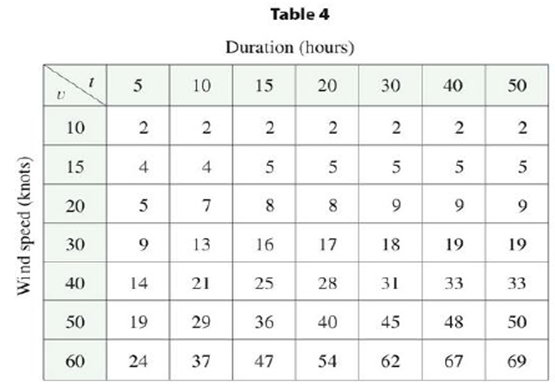Chapter 14.1, Problem 7E

Chapter
Section
Textbook Problem

The wave heights h in the open sea depend on the speed v of the wind and the length of time t that the wind has been blowing at that speed. Values of the function h = f(v, I) are recorded in feet in Table 4.(a) What is the value of f(40, 15)? What is its meaning?(b) What is die meaning of the function h = f(30, t)? Describe the behavior of this function.(c) What is the meaning of the function h = f(v, 30)? Describe the behavior of this function.(a)

To determine

To find: The value of f(40,15) and interpret the meaning.

Explanation

It is observed from the given Table that the corresponding value of f(40,50)

(b)

To determine

To explain: The meaning of the function h=f(80,h) and behavior of this function.

(c)

To determine

To explain: The meaning of the function h=f(v,30) and behavior of this function.

Still sussing out bartleby?

Check out a sample textbook solution.

See a sample solution

The Solution to Your Study Problems

Bartleby provides explanations to thousands of textbook problems written by our experts, many with advanced degrees!

Get Started

In Exercises 7-12, solve for y in terms of x. (y3)2=5+(x+1)2

Calculus: An Applied Approach (MindTap Course List)

23(4x2x21)+3(33x1)

Applied Calculus for the Managerial, Life, and Social Sciences: A Brief Approach

In Problems 35-42, simplify each complex fraction. 40.

Mathematical Applications for the Management, Life, and Social Sciences

Factor completely. 2x23x+1

Trigonometry (MindTap Course List)

limx1x3x21x = _____. a) 1 b) 1 c) 0 d) does not exist

Study Guide for Stewart's Single Variable Calculus: Early Transcendentals, 8th

0 1 π It does not exist.

Study Guide for Stewart's Multivariable Calculus, 8th